Quantity: 0

Total: 0,00

# Cylindrical solids

### Cylindrical solids

This animation demonstrates various types of cylindrical solids as well as their lateral surfaces.

Mathematics

Keywords

cylindrical solid, right circular cylinder, oblique circular cylinder, right prism, right rectangular prism, lateral surface, base circle, rectangle, solid geometry, mathematics

### Related items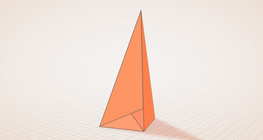#### Császár polyhedron

The Császár polyhedron is a nonconvex polyhedron with 14 triangular faces.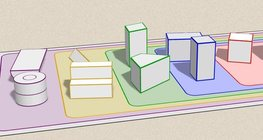#### Grouping of solids

This animation demonstrates various groups of solids through examples.#### Grouping of solids 1

This animation demonstrates various groups of solids through examples.#### Grouping of solids 2

This animation demonstrates various groups of solids through examples.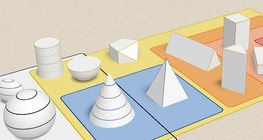#### Grouping of solids 3

This animation demonstrates various groups of solids through examples.#### Grouping of solids 4

This animation demonstrates various groups of solids through examples.#### Perimeter, area, surface area and volume

This animation presents the formulas to calculate the perimeter and area of shapes as well as the surface area and volume of solids.#### Platonic solids

This animation demonstrates the five regular three-dimensional (or Platonic) solids, the best known of which is the cube.#### Szilassi polyhedron

This special concave polyhedron was named after a Hungarian mathematician.#### Solids of revolution

Rotating a geometric shape around a line within its geometric plane as an axis results in a solid of revolution.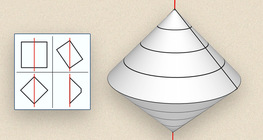#### Solids of revolution (exercise)

An exercise about the generation of solids of revolution.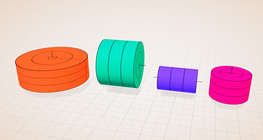#### Solids of revolution (rectangle)

Rotating a rectangle around its axes of symmetry or around its sides results in solids of revolution.#### Sphere

A sphere is the set of points which are all within the same distance from a given point in space.#### Conic solids

This animation demonstrates various types of cones and pyramids.